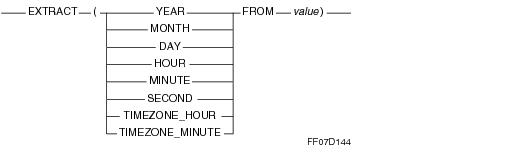# 15.00 - EXTRACT - Teradata Database

## Teradata Database SQL Functions, Operators, Expressions, and Predicates

Product
Release Number
15.00
Content Type
Programming Reference
Publication ID
B035-1145-015K
Language
English (United States)

## EXTRACT

### Purpose

Extracts a single specified full ANSI SQL:2011 field from any DateTime or Interval value, converting it to an exact numeric value.

### Syntaxwhere:

 Syntax element … Specifies … YEAR that the integer value for YEAR is to be extracted from the date represented by value. MONTH that the integer value for MONTH is to be extracted from the date represented by value. DAY that the integer value for DAY is to be extracted from the date represented by value. HOUR that the integer value for HOUR is to be extracted from the date represented by value. MINUTE that the integer value for MINUTE is to be extracted from the date represented by value. TIMEZONE_HOUR that the integer value for TIMEZONE_HOUR is to be extracted from the date represented by value. TIMEZONE_MINUTE that the integer value for TIMEZONE_MINUTE is to be extracted from the date represented by value. SECOND that both the integer and decimal portions value for SECOND are to be extracted from the date represented by value. The returned value has a data type of DECIMAL(8,2). value an expression that results in a DateTime, Interval, or UDT value.

### ANSI Compliance

EXTRACT is partially ANSI SQL:2011 compliant.

ANSI SQL:2011 EXTRACT allows extraction of any field in any DateTime or Interval value. In addition to the ANSI SQL:2011 extract function, Teradata SQL also supports HOUR, MINUTE, or SECOND extracted from a floating point value.

### Arguments

 IF value is … THEN … a character string expression that represents a date the string must match the 'YYYY-MM-DD' format. a character string expression that represents a time the string must match the 'HH:MI:SS.SSSSSS' format. a floating point type value must be a time value encoded with the algorithm HOUR * 10000 + MINUTE * 100 + SECOND. Only HOUR, MINUTE, and SECOND can be extracted from a floating point value. Externally created time values can be appropriately encoded and stored in a REAL column to any desired precision if the encoding creates a value representable by REAL without precision loss. Do not store time values as REAL in any new applications. Instead, use the more rigorously defined ANSI SQL:2011 DateTime data types. a UDT the UDT must have an implicit cast that casts between the UDT and any of the following predefined types: Numeric Character DateTime To define an implicit cast for a UDT, use the CREATE CAST statement and specify the AS ASSIGNMENT clause. For more information on CREATE CAST, see SQL Data Definition Language. Implicit type conversion of UDTs for system operators and functions, including EXTRACT, is a Teradata extension to the ANSI SQL standard. To disable this extension, set the DisableUDTImplCastForSysFuncOp field of the DBS Control Record to TRUE. For details, see Utilities: Volume 1 (A-K). For more information on implicit type conversion of UDTs, see “Implicit Type Conversions” on page 583. not a character string expression or floating point type or UDT the expression must evaluate to a DateTime or Interval type.

### Results

EXTRACT returns an exact numeric value for ANSI SQL:2011 DateTime values.

EXTRACT returns values adjusted for the appropriate time zone if the data type of the argument is TIME or TIMESTAMP.

If no time zone is specified for the argument, then the time zone displacement based on the current session time zone is used; otherwise, the explicit time zone of the argument is used. You can use the AT clause to explicitly specify a time zone for the argument. For details, see “ANSI DateTime Expressions” on page 826.

If you extract SECOND, then if the value has a seconds fraction precision of zero, the result is integer; if the value has a seconds factional prevision of greater than zero, the result is DECIMAL with the scaling as specified for the SECOND field in its data description.

If you extract anything else, the result is INTEGER with 32 bits of precision.

 If you extract … THEN … SECOND If value has a seconds fractional of precision of: zero, the result is INTEGER. greater than zero, the result is DECIMAL with the scaling as specified for the SECOND field in its data description. anything else the result is INTEGER, with 32 bits of precision.

If value is NULL, the result is NULL.

### Example

The following example returns the year, as an integer, from the current date.

`   SELECT EXTRACT (YEAR FROM CURRENT_DATE);`

### Example

Assuming PurchaseDate is a DATE field, this example returns the month of the date value formed by adding 90 days to PurchaseDate as an integer.

`   SELECT EXTRACT (MONTH FROM PurchaseDate+90) FROM SalesTable;`

### Example

The following returns 12 as an integer.

`   SELECT EXTRACT (DAY FROM '1996-12-12');`

### Example

This example returns an error because the character literal does not evaluate to a valid date.

`   SELECT EXTRACT (DAY FROM '1996-02-30');`

### Example

The following returns an error because the character string literal does not match the ANSI SQL:2011 date format.

`   SELECT EXTRACT (DAY FROM '96-02-15');`

If the argument to EXTRACT is a value of type DATE, the value contained is warranted to be a valid date, for which EXTRACT cannot return an error.

### Example

The following example relates to non-ANSI DateTime definitions. If the argument is a character literal formatted as a time value, it is converted to REAL and processed. In this example, 59 is returned.

`   SELECT EXTRACT (MINUTE FROM '23:59:17.3');`

### Example

This example returns the hour, as an integer, from the current time.

`   SELECT EXTRACT (HOUR FROM CURRENT_TIME);`

Current time is retrieved as the system value TIME, to the indicated precision.

### Example

The following example returns the seconds as DECIMAL(8,2). This is based on the fractional seconds precision of 2 for CURRENT_TIME.

`   SELECT EXTRACT (SECOND FROM CURRENT_TIME (2));`